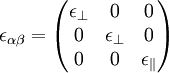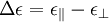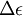2022: SklogWiki celebrates 15 years on-line

# Nematic phase

The nematic phase of a liquid crystal has orientational order, but no positional order .

## Dielectric tensor

In the uniaxial nematic phase, defining the z-axis to be parallel to the nematic axis, one has$\epsilon_{\alpha \beta}= \begin{pmatrix} \epsilon_\bot & 0 & 0 \\ 0 & \epsilon_\bot & 0 \\ 0 & 0 & \epsilon_\| \end{pmatrix}$

The anisotropy is defined as$\Delta \epsilon = \epsilon_\| - \epsilon_\bot$.

The response of a nematic liquid crystal to an external electric field depends on both the sign and the magnitude of$\Delta \epsilon$.

## Chiral nematic phase

See Chiral phases.